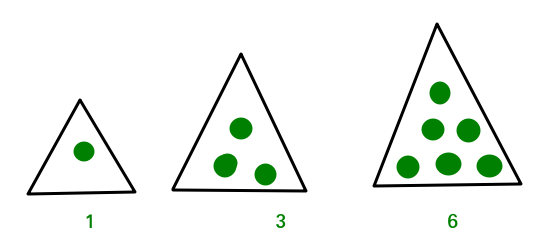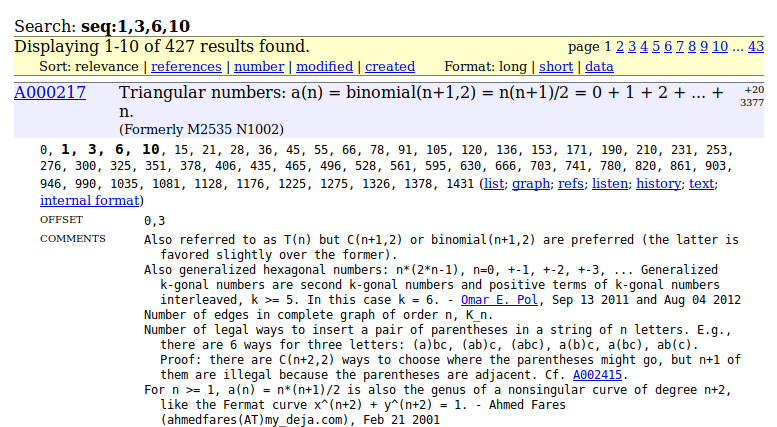How to use OESIS (On-line Encyclopedia of Integer Sequences)

• Difficulty Level : Medium
• Last Updated : 10 Feb, 2018

We sometimes land up in a situation when various coding problems can be simplified to a mathematical formula but often finding that formula isn’t that straightforward .Here comes, OESIS for rescue. We can calculate the terms for initial indices i.e n=0,1,2,3,…….. and then may use OEIS to find the mathematical expression.

This article focuses on using OEIS (On-line Encyclopedia of Integer Sequences) to solve many complex problems easily by formulating them in some sort of mathematical expression. OEIS is a massive encyclopedia where we can look for various sequences and find their mathematical expression,examples and related facts about them .

Lets try to understand using an example: You are given n balls and are supposed to make equilateral triangle as shown in the image below.Each subsequent pattern should add a new row  to the triangle.Now if we see the sequence of dots being added to form triangle,

The first few numbers are 1, 3, 6, 10, 15

This problem is known as  triangular number which counts the objects that can form an equilateral triangle,. The nth triangular number is the number of dots composing a triangle with n dots on a side, and is equal to the sum of the n natural numbers from 1 to n. The sequence of triangular numbers starting at the 0th triangular number, is:

0, 1, 3, 6, 10, 15 ….

Now we have got the sequence and if we search it on OEIS ,we get the following result:OESIS proves extremely helpful in finding the algorithm to solve the problem.OEIS records information on integer sequences of interest to both professional mathematicians and amateurs, and is widely cited. As of 22 August 2015 it contains over 260,000 sequences, making it the largest database of its kind.

This article is contributed by Siddharth Lalwani. If you like GeeksforGeeks and would like to contribute, you can also write an article and mail your article to contribute@geeksforgeeks.org. See your article appearing on the GeeksforGeeks main page and help other Geeks.